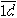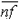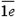You may also likeDouble Digit

Choose two digits and arrange them to make two double-digit numbers. Now add your double-digit numbers. Now add your single digit numbers. Divide your double-digit answer by your single-digit answer. Try lots of examples. What happens? Can you explain it?Repeaters

Choose any 3 digits and make a 6 digit number by repeating the 3 digits in the same order (e.g. 594594). Explain why whatever digits you choose the number will always be divisible by 7, 11 and 13.Digit Sum

What is the sum of all the digits in all the integers from one to one million?

Tis Unique

Age 11 to 14 Challenge Level:

Congratulations to all the following for their correct solutions:

Andrei Lazanu Lazanu (School number 205, Bucharest),
Fiona Watson (Stamford High School),
Musab Khawaja,
Chong Ching Tong, Chen Wei Jian and Teo Seow Tian (River Valley High School, Singapore),
Clement Goh Tian (River Valley High School, Singapore),
Emma Holmes

It is good to see you explain how you came to your answer. Some of you said you solved the problem by "trial and error" but I am sure you made some decisions along the way. Sometimes it is necessary to try several possible numbers before finding the one that works for other constraints. Next time try to explain your thinking. I have included Andrei's solution as one of those that gave a very complete explanation of how they thought the problem through.

First I observed that the first digit of the result must be 1:

 * * 4 + 2 8 * _ _ _ _ 1 * * *

I used for the other letters the following notation:

 a b 4 + 2 8 c _ _ _ _ 1 d e f

2 + a + n =, where n can be 0 or 1.
a + n = 8 + d, where a and d are smaller than 10.

 1.1. n = 0

a = 8 + d.
a can be 8 or 9 and d 0 and 1 respectively, but in the first case 8 was used, and in the second case 0 was used.

 1.2. n = 1

a + 1 = 8 + d
a can be 7, 8 and 9 and d can be 0, 1 and 2. In the first situation it works because the digits weren't used another time, in the second combination it doesn't work, and the last situation doesn't work. The result is:

 7 b 4 + 2 8 c _ _ _ _ 1 0 e f

4 + c =c can be : 3, 5, 6, 9. Thenis: 7, 9, 10, 13. The possibilities are:

c = 5; f = 9

c = 9; f = 3

 2.1. c = 5; f = 9

 7 b 4 + 2 8 5 _ _ _ _ 1 0 e 9

b + 8 =Using only 3 and 6 there isn't any possibility.

 2.2. c = 9; f = 3

 7 b 4 + 2 8 9 _ _ _ _ 1 0 e 3

b + 8 + 1 =b + 9 =In this situation only b = 6 and e = 5 satisfies the condition.

This is the only solution for the problem, because using a step-by-step method, I obtained only one solution.